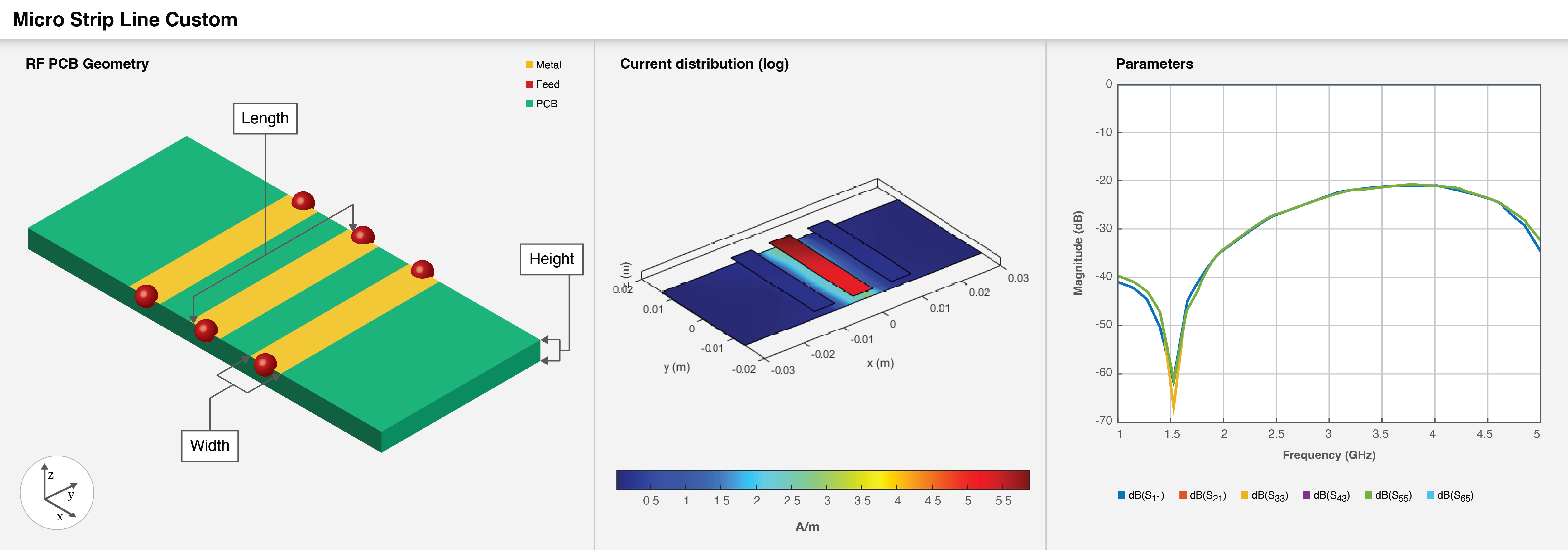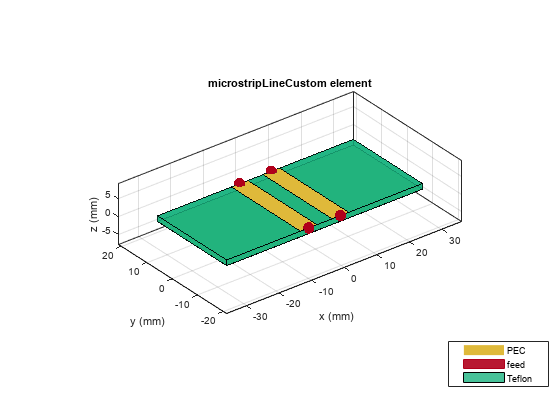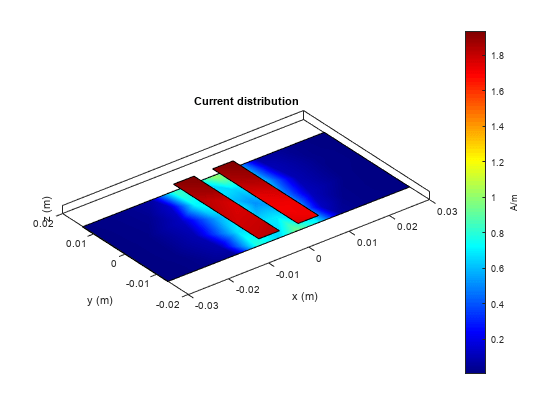# microstripLineCustom

Create coupled form of single or differential microstrip transmission line

Since R2022b

## Description

Use the `microstripLineCustom` object to create a coupled form of single or differential microstrip transmission line. A microstrip line is a transmission line that is a basic building block for most RF planar microwave devices. You can use this transmission line to connect two PCB components or to create components such as filters, couplers, and feeding elements of several types of antennas.A few applications of microstrip transmission lines are:

• Creating matching feed and coupling networks

• Transmitting power from one component to another

• Feeding planar antennas and coupling structures

• Creating varying inductances or capacitances using open- or short ended- transmission lines

## Creation

### Syntax

``microstrip = microstripLineCustom``
``microstrip = microstripLineCustom(Name=Value)``

### Description

````microstrip = microstripLineCustom` creates a coupled form of single or differential microstrip transmission line. The default properties are for a design frequency of 2.5 GHz.```

example

````microstrip = microstripLineCustom(Name=Value)` sets properties using one or more name value pair arguments. For example, ```microstrip = microstripLineCustom(TraceSpacing=0.0300)``` creates a custom differential microstrip transmission line with a trace spacing of 0.0300 meters. Properties not specified retain their default values.```

## Properties

expand all

Types of traces, specified as `'Single'` or `'Differential'`.

Example: ```microstrip = microstripLineCustom(TraceType='Differential')```

Data Types: `string` | `char`

Length of the trace in meters, specified as a positive scalar.

Example: `microstrip = microstripLineCustom(TraceLength=0.0371)`

Data Types: `double`

Width of the trace in meters, specified as a positive scalar.

Example: `microstrip = microstripLineCustom(TraceWidth=0.0041)`

Data Types: `double`

Spacing between the two traces of a differential pair in meters, specified as a positive scalar. Set the `TraceType` property to `'Differential'` to enable this property.

Example: `microstrip = microstripLineCustom(TraceSpacing=0.0041)`

Data Types: `double`

Position to offset reference trace along the X-axis, specified as a positive scalar.

Example: `microstrip = microstripLineCustom(TraceOffsetX=0.5)`

Data Types: `double`

Height from the custom microstrip line to the ground plane in meters, specified as a positive scalar.

In the case of a multilayer substrate, you can use the height property to create a microstrip line at the interface of the two dielectrics.

Example: `microstrip = microstripLineCustom(Height=0.0026)`

Data Types: `double`

Length of the ground plane in meters, specified as a positive scalar.

Example: ```microstrip = microstripLineCustom(GroundPlaneLength=0.0500)```

Data Types: `double`

Gap between the left coupled traces in meters, specified as a scalar or vector. Set this property to `0`, if there are no left coupled traces.

Example: ```microstrip = microstripLineCustom(LeftCoupledTraceGap=0)```

Data Types: `double`

Gap between the right coupled traces in meters, specified as a scalar or vector. Set this property to `0`, if there are no right coupled traces.

Example: ```microstrip = microstripLineCustom(RightCoupledTraceGap=0)```

Data Types: `double`

Type of dielectric material used as a substrate, specified as a `dielectric` object. The dielectric material in a `microstriplineCustom` with default properties is Teflon. The thickness of the default dielectric material Teflon is `0.0016` m or the same as the height property.

Example: `d = dielectric('FR4');` `microstrip = microstripLineCustom(Substrate=d)`

Type of metal used in conducting layers, specified as a `metal` object. The type of metal in a `microstriplineCustom` object with default properties is PEC.

Example: `m = metal('PEC');` `microstrip = microstripLineCustom(Conductor=m)`

## Object Functions

 `charge` Calculate and plot charge distribution `current` Calculate and plot current distribution `design` Design microstrip transmission line around specified frequency `feedCurrent` Calculate current at feed port `getZ0` Calculate characteristic impedance of transmission line `layout` Plot all metal layers and board shape `mesh` Change and view mesh properties of metal or dielectric in PCB component `shapes` Extract all metal layer shapes of PCB component `show` Display PCB component structure or PCB shape `sparameters` Calculate S-parameters for RF PCB objects

## Examples

collapse all

Create a coupled form of differential microstrip line with two pairs of right coupled lines.

```microstrip = microstripLineCustom(TraceType='Differential',TraceWidth=... 0.002,TraceSpacing=0.0005,RightCoupledTraceGap=[0.003,0.003],... LeftCoupledTraceGap=0);```

View the custom transmission line.

`show(microstrip)`Create a differential microstrip transmission line with no left or right traces

```microstrip = microstripLineCustom(TraceType='Differential',TraceSpacing=... 0.0046,LeftCoupledTraceGap=0,RightCoupledTraceGap=0);```

View the transmission line.

`show(microstrip)`Set the voltage on the transmission line ports. View the layout.

```v = voltagePort(4); v.FeedVoltage = [1 0 1 0]; v.FeedPhase = [0 0 180 0]; figure; layout(microstrip)```Analyze the current at 2.5 GHz.

```figure; current(microstrip,2.5e9,Excitation=v)```Pozar, David M. Microwave Engineering. 4th ed. Hoboken, NJ: Wiley, 2012.